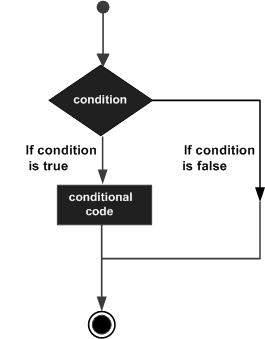Python Decision Making - Syntax and Examples | W3school

In decision making specific actions are predefined according to the anticipation of certain conditions. Multiple conditions and expressions are evaluated to give TRUE or FALSE as the outcome. Based on the outcome the executable action is decided by the code.

A standard decision-making structure used in almost all the programming languages is shown below:Any non-null or non-zero value us assumed to be TRUE in Python language. If the value is null or zero then it is assumed as FALSE.

Following types of decision-making statements can be used in Python:

1.      If statement: The if statement makes a decision based on the output of the logical expression that compares data and returns a TRUE or a FALSE. If TRUE, then the block of code corresponding to the if statement is executed. For example:

var1 = 100

if var1:

print “1 – Got a true expression value”

print var1

var2 = 0

if var2:

print “2 – Got a true expression value”

print var2

The above code gives the below output:

1 – Got a true expression value

100

2.      If…else statements: The else statement block is executed if the logical expression defined in if statement returns a FALSE value. For example:

var1 = 100

if var1:

print “1 – Got a true expression value”

print var1

else:

print “1 – Got a false expression value”

print var1

var2 = 0

if var2:

print “2 – Got a true expression value”

print var2

else:

print “2 – Got a false expression value”

print var2

The above set of code gives the below output:

1 – Got a true expression value

100

2 – Got a false expression value

0

3.      Nested if statements: If another condition has to be checked after a certain condition then nested if statements can be used. In a nested, if construct, the if…elif…else construct is used to execute statements based on multiple conditions. For example:

var = 100

if var < 200:

print “Expression value is less than 200”

if var == 150:

print “Which is 150”

elif var == 100:

print “Which is 100”

elif var == 50:

print “Which is 50”

elif var < 50:

print “Expression value is less than 50”

else:

print “Could not find the true expression”